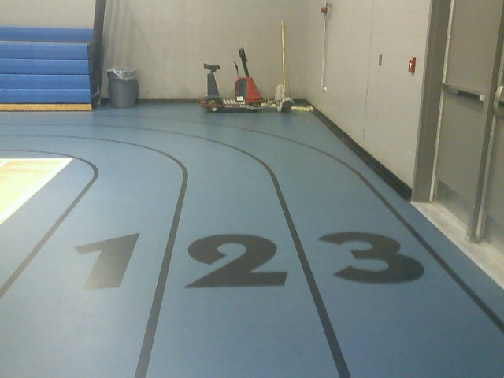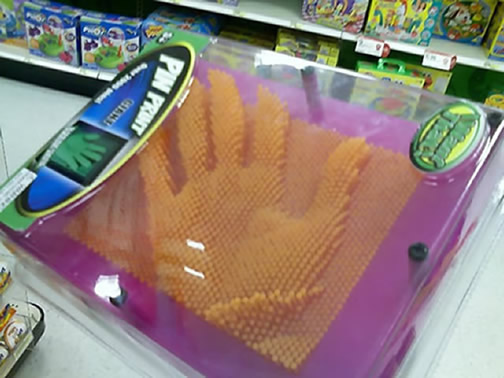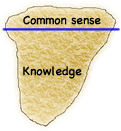Processes for the practice of mathematics

Problem solving Tools to facilitate mathematical problem solving

• Problem solving Concepts, misconceptions, & outcomes by levels in the mathematical knowledge base

Reasoning and proof

• Reasoning & proof Concepts, misconceptions, & outcomes by levels in the mathematical knowledge base

Representation

Representation Concepts, misconceptions, & outcomes by levels in the mathematical knowledge base

Area representations

Lynne Outhred and Michael Mitchelmore asked learners to illustrate the area of a rectangular shape. They found learners demonstrated four different strategies: incomplete covering, visual covering, concrete covering, and measurement. The drawings below show samples from each.Representation of relative position of objectsIllustration of Internal RepresentationActual object, real world, or external representation

Representation of external objects with points on an other external objectWhat does this have to do with mathematical representations?

Communication

Communication Concepts, misconceptions, & outcomes by levels in the mathematical knowledge base

Connections & perspective

Connections & perspective Concepts, misconceptions, & outcomes by levels in the mathematical knowledge base

Information to facilitate literacy in connections & perspective & tools to achieve it

• Mathematical ideas build upon each other.
• Mathematical ideas are connected to other mathematical ideas.
• Mathematical ideas are connected to the world.

Activities to facilitate reasoning & proof

Other math knowlege base &

activity pages

Content dimensions

Common sense & knowledge1. WIn
2. NOnBA
3. THABTHIn
4. DUBA
5. SATOn
6. SHAPE
7. PETHOnBA
8. PETHABTHIn
9. SHOnKA
10. GTHEBOn

Interesting bookThe Power of Logical Thinking: Easy Lessons in the Art of Reasoning... and Hard Facts About Its Absence in Our Lives, Marilyn Vos Savant (1996) ISBN 0-312-13985-3 Saint Martin's Press: New York.